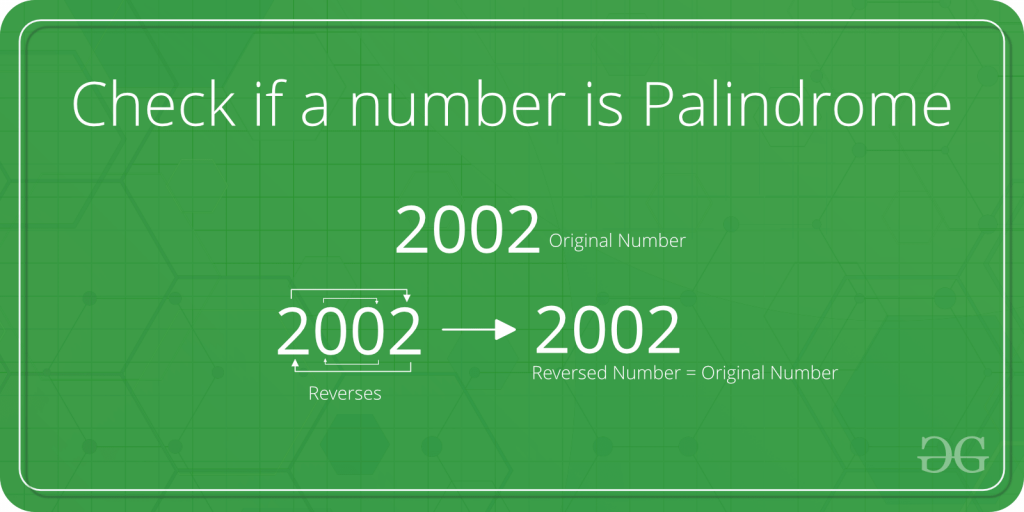Skip to content
Related Articles
Program to check the number is Palindrome or not
• Difficulty Level : Basic
• Last Updated : 22 Mar, 2021

Given an integer N, write a program that returns true if the given number is a palindrome, else return false.
Examples:

```Input: N = 2002
Output: true

Input: N = 1234
Output: false```Approach:
A simple method for this problem is to first reverse digits of n, then compare the reverse of n with n. If both are same, then return true, else false.
Below is the implementation of the above approach:

## C++

 `// C program to check whether a number``// is Palindrome or not.` `#include ` `/* Iterative function to reverse digits of num*/``int` `reverseDigits(``int` `num)``{``    ``int` `rev_num = 0;``    ``while` `(num > 0) {``        ``rev_num = rev_num * 10 + num % 10;``        ``num = num / 10;``    ``}``    ``return` `rev_num;``}` `/* Function to check if n is Palindrome*/``int` `isPalindrome(``int` `n)``{` `    ``// get the reverse of n``    ``int` `rev_n = reverseDigits(n);` `    ``// Check if rev_n and n are same or not.``    ``if` `(rev_n == n)``        ``return` `1;``    ``else``        ``return` `0;``}` `/*Driver program to test reversDigits*/``int` `main()``{``    ``int` `n = 4562;``    ``printf``(``"Is %d a Palindrome number? -> %s\n"``, n,``           ``isPalindrome(n) == 1 ? ``"true"` `: ``"false"``);` `    ``n = 2002;``    ``printf``(``"Is %d a Palindrome number? -> %s\n"``, n,``           ``isPalindrome(n) == 1 ? ``"true"` `: ``"false"``);``    ``return` `0;``}`

## Java

 `// Java program to check whether a number``// is Palindrome or not.` `class` `GFG``{``    ``/* Iterative function to reverse digits of num*/``    ``static` `int` `reverseDigits(``int` `num)``    ``{``        ``int` `rev_num = ``0``;``        ``while` `(num > ``0``) {``            ``rev_num = rev_num * ``10` `+ num % ``10``;``            ``num = num / ``10``;``        ``}``        ``return` `rev_num;``    ``}``    ` `    ``/* Function to check if n is Palindrome*/``    ``static` `int` `isPalindrome(``int` `n)``    ``{``    ` `        ``// get the reverse of n``        ``int` `rev_n = reverseDigits(n);``    ` `        ``// Check if rev_n and n are same or not.``        ``if` `(rev_n == n)``            ``return` `1``;``        ``else``            ``return` `0``;``    ``}``    ` `    ``/*Driver program to test reversDigits*/``    ``public` `static` `void`  `main(String []args)``    ``{``        ``int` `n = ``4562``;``        ``System.out.println(``"Is"` `+ n + ``"a Palindrome number? -> "` `+``            ``(isPalindrome(n) == ``1` `? ``"true"` `: ``"false"``));``    ` `        ``n = ``2002``;``        ` `        ``System.out.println(``"Is"` `+ n + ``"a Palindrome number? -> "` `+``            ``(isPalindrome(n) == ``1` `? ``"true"` `: ``"false"``));` `    ``}` `}` `// This code is contributed``// by Hritik Raj ( ihritik )`

## Python3

 `# Python3 program to check whether a``# number is Palindrome or not.` `# Iterative function to reverse``# digits of num``def` `reverseDigits(num) :` `    ``rev_num ``=` `0``;``    ``while` `(num > ``0``) :``        ``rev_num ``=` `rev_num ``*` `10` `+` `num ``%` `10``        ``num ``=` `num ``/``/` `10``    ` `    ``return` `rev_num` `# Function to check if n is Palindrome``def` `isPalindrome(n) :` `    ``# get the reverse of n``    ``rev_n ``=` `reverseDigits(n);` `    ``# Check if rev_n and n are same or not.``    ``if` `(rev_n ``=``=` `n) :``        ``return` `1``    ``else` `:``        ``return` `0` `# Driver Code``if` `__name__ ``=``=` `"__main__"` `:` `    ``n ``=` `4562``    ` `    ``if` `isPalindrome(n) ``=``=` `1` `:``        ``print``(``"Is"``, n, ``"a Palindrome number? ->"``, ``True``)``        ` `    ``else` `:``        ``print``(``"Is"``, n, ``"a Palindrome number? ->"``, ``False``)` `    ``n ``=` `2002``    ` `    ``if` `isPalindrome(n) ``=``=` `1` `:``        ``print``(``"Is"``, n, ``"a Palindrome number? ->"``, ``True``)``        ` `    ``else` `:``        ``print``(``"Is"``, n, ``"a Palindrome number? ->"``, ``False``)` `# This code is contributed by Ryuga`

## C#

 `// C# program to check whether a number``// is Palindrome or not.` `using` `System;``class` `GFG``{``    ``/* Iterative function to reverse digits of num*/``    ``static` `int` `reverseDigits(``int` `num)``    ``{``        ``int` `rev_num = 0;``        ``while` `(num > 0) {``            ``rev_num = rev_num * 10 + num % 10;``            ``num = num / 10;``        ``}``        ``return` `rev_num;``    ``}``    ` `    ``/* Function to check if n is Palindrome*/``    ``static` `int` `isPalindrome(``int` `n)``    ``{``    ` `        ``// get the reverse of n``        ``int` `rev_n = reverseDigits(n);``    ` `        ``// Check if rev_n and n are same or not.``        ``if` `(rev_n == n)``            ``return` `1;``        ``else``            ``return` `0;``    ``}``    ` `    ``/*Driver program to test reversDigits*/``    ``public` `static` `void`  `Main()``    ``{``        ``int` `n = 4562;``        ``Console.WriteLine(``"Is"` `+ n + ``"a Palindrome number? -> "` `+``            ``(isPalindrome(n) == 1 ? ``"true"` `: ``"false"``));``    ` `        ``n = 2002;``        ` `        ``Console.WriteLine(``"Is"` `+ n + ``"a Palindrome number? -> "` `+``            ``(isPalindrome(n) == 1 ? ``"true"` `: ``"false"``));` `    ``}` `}` `// This code is contributed``// by Hritik Raj ( ihritik )`

## PHP

 ` 0)``    ``{``        ``\$rev_num` `= ``\$rev_num` `* 10 +``                   ``\$num` `% 10;``        ``\$num` `= ``\$num` `/ 10;``    ``}``    ``return` `\$rev_num``;``}` `// Function to check if n is Palindrome``function` `isPalindrome(``\$n``)``{` `    ``// get the reverse of n``    ``\$rev_n` `= reverseDigits(``\$n``);` `    ``// Check if rev_n and n are same or not.``    ``if` `(``\$rev_n` `== ``\$n``)``        ``return` `1;``    ``else``        ``return` `0;``}` `// Driver Code``\$n` `= 4562;``echo` `"Is "``, ``\$n` `, ``" a Palindrome number? ->"``;` `if``(isPalindrome(``\$n``) == 1)``    ``echo` `"true"` `;``else``    ``echo` `"false"``;``echo` `"\n"``;` `\$n` `= 2002;``echo` `"Is "``, ``\$n` `, ``" a Palindrome number? ->"``;``if``(isPalindrome(!``\$n``))``    ``echo` `"true"` `;``else``    ``echo` `"false"``;` `// This code is contributed by jit_t``?>`

## Javascript

 ``
Output:
```Is 4562 a Palindrome number? -> false
Is 2002 a Palindrome number? -> true```

Attention reader! Don’t stop learning now. Get hold of all the important DSA concepts with the DSA Self Paced Course at a student-friendly price and become industry ready.  To complete your preparation from learning a language to DS Algo and many more,  please refer Complete Interview Preparation Course.

In case you wish to attend live classes with industry experts, please refer Geeks Classes Live

My Personal Notes arrow_drop_up